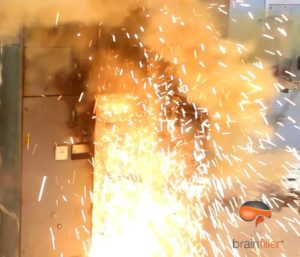# Zero-Sequence Impedance and Incident EnergyA question that I am often asked either on-line or in one of my arc flash training classes is in regards to incident energy calculations and line-ground short circuit current:

“Since it is possible for the line-to-ground short circuit current to be greater than the three- phase current, could the line-to-ground condition be the worst case for incident energy calculations using IEEE 1584 equations?”

The longer answer: Let’s look at the equations for each short circuit calculation using symmetrical components.

The equation for calculating the three-phase fault current is:

I(3phase) = V/Z1

The equation for calculating the line-to-ground fault current is:

I(line-ground) 3V / (Z1+ Z2+ Z0)

Where:

V = voltage
Z1 = Positive sequence impedance
Z2 = Negative sequence impedance (Z2 is normally equal to Z1)
Z0 = Zero sequence impedance
I(3phase) = Three Phase Short Circuit Current
I(line-ground) = Line-to-Ground Short Circuit Current

All values are in “per unit”

For a frame of reference, if Z1 and Z2 are equal and  Z0 is also equal (which is not too common)

Then Z1 = Z2 = Z0

For this condition the calculated line-to-ground short circuit current would be:

I(line-ground) = 3V / (Z1+ Z2+ Z0) = 3V / 3Z = V/ Z1

In other words, the line-to-ground short circuit current would be the same magnitude as the three-phase case for this specific condition.

However, for grounded systems Z0 is normally greater than Z1 and Z2.   With Z0 normally being larger than Z1 and Z2, the resulting line-to-ground short circuit current is normally less than the three-phase case. For the case of ungrounded systems where ground fault current does not flow, Z0 is infinite.

As mentioned, it is also possible for Z0 to be slightly lower than Z1 and Z2 resulting in the line-to-ground short circuit current being slightly larger than the three-phase current. One case where this can occur is at or near the secondary side of a delta-wye transformer.  This can occur near some generators as well.  In practical terms, this means the calculated short circuit current for a line-to-ground fault would be slightly higher than for a three-phase fault for this case.

So back to the original question.  If the line-ground short circuit current is slightly higher than the three-phase case, would the line-ground case result in the worst-case calculated incident energy?

Elaborating on the “No” answer, calculations using the IEEE 1584 equations are based on a three-phase arc flash i.e. all three phases contributing to the incident energy. Even if the line-ground short circuit current is higher, the incident energy is only for one phase.  The slightly lower three-phase current involves incident energy from all three phases so it would be the worst case.

For more about short circuit current calculations and symmetrical components, check out my short video.